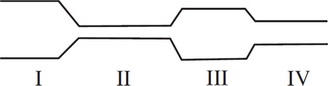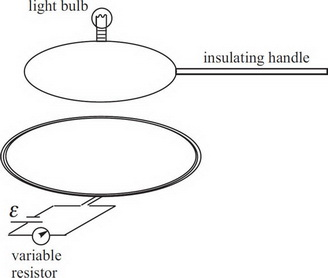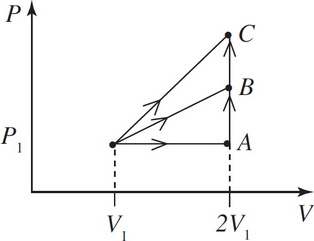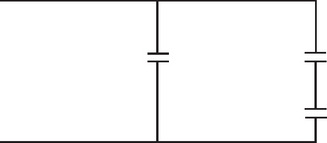# AP Physics 2 Practice Test 5

### Test Information10 questions18 minutes

1.A fluid flows through a pipe of changing cross sections as shown. In which section would the pressure of the fluid be greatest?

2.In the figure shown above, a loop of metal wire containing a tiny light bulb is attached to an insulating handle and placed over a coil of wire in which a current can be established by a source of emf and controlled by a variable resistor. The plane of the top loop is parallel to the plane of the bottom coil. Which of the following could NOT cause the bulb to light?

3. Which of the following types of electromagnetic radiation has the longest wavelength?

4. Which of the following statements about images is true?

5. A fluid of density ρ flows through a horizontal pipe with negligible viscosity. The flow is streamlined with constant flow rate. The diameter of the pipe at Point 1 is d and the flow speed is V. If the diameter of the pipe at Point 2 is d/3, then the pressure at Point 2 is

6. A charged particle moves through a magnetic field and experiences a force f. New particles are sent into the same magnetic field. If the new particles have twice the charge, twice the mass, and twice the velocity, the new force would be

7.A cylinder with a piston on top contains an ideal gas at an initial pressure P1, volume v1, and Temperature T1. The gas is allowed to expand to twice its original volume in three different ways as shown. The mechanical work done by the gas is

8.In the figure above, each capacitor has a capacitance of C. What is the overall capacitance of the above circuit?

9. You have a wire of length L, radius Q, and resistance Q. You need to obtain half that resistance using the same material and changing only one factor. You could

10. An electric motor has a label on it that reads: Input: 120V AC, 1.0 Amps, 60 Hz - Efficiency - 75%. At what constant speed can the motor lift up a 6 kg mass?